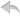注册    登录创作新主题

Python
 python开源   Django   Python   DjangoApp   pycharm
DATA
 docker   Elasticsearch

 问与答   闲聊   招聘   翻译   创业   分享发现   分享创造   求职   区块链   支付之战
WEB开发
 linux   MongoDB   Redis   DATABASE   NGINX   其他Web框架   web工具   zookeeper   tornado   NoSql   Bootstrap   js   peewee   Git   bottle   IE   MQ   Jquery

Python88.com
 反馈   公告   社区推广

Python社区  »  Python

# 用Python中纬度计算地球半径-复制公式

Kah • 6 月前 • 66 次点击

# 示例代码

``````import math

a = 6378.137  #Radius at sea level at equator
b = 6356.752  #Radius at poles

c = (a**2*math.cos(B))**2
d = (b**2*math.sin(B))**2
e = (a*math.cos(B))**2
f = (b*math.sin(B))**2

R = math.sqrt((c+d)/(e+f))

return R
``````

Python社区是高质量的Python/Django开发社区•   1 楼 foxpal    12 月前 那是因为 ``` math.cos ``` 和 ``` math.sin ``` 以弧度表示。您需要将函数顶部的度数转换为弧度: ``````B *= math.pi/180 ``````•   2 楼 nikhildr22    12 月前 python数学库将弧度作为三角函数的输入, 所以一定要把B的值转换成弧度 它可以通过 ``` B=math.radians(B) ``` 最终代码: ``````import math def radius (B): B=math.radians(B) #converting into radians a = 6378.137 #Radius at sea level at equator b = 6356.752 #Radius at poles c = (a**2*math.cos(B))**2 d = (b**2*math.sin(B))**2 e = (a*math.cos(B))**2 f = (b*math.sin(B))**2 R = math.sqrt((c+d)/(e+f)) return R ``````